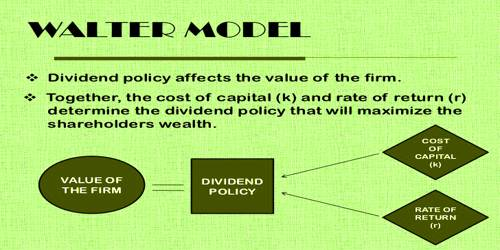# Assumptions of Walter’s theory of Dividend PolicyAssumptions of Walter’s theory of Dividend Policy

Dividend policy means how much dividend would as a retained in a company and how much distribute to stockholders. All these related activities of the dividend are called dividend policy.

Walter’s theory on dividend policy believes in the relevance concept of the dividend. According to this concept, the dividend decision of the company affects its valuation. The companies paying higher dividends have more value as compared to the companies that pay lower dividends or do not pay at all. Walter’s theory further explains this concept in a mathematical model.

According to this concept, the dividend decision of the company affects its valuation. His model shows clearly the importance of the relationship between the firm’s internal rate of return (r) and its cost of capital (k) in determining the dividend policy that will maximize the wealth of shareholders.

Walter’s formula to calculate the market price per share (P) is:

P = D/k {r*(E-D)/k}/k, where

• P = market price per share
• D = dividend per share
• E = earnings per share
• r = internal rate of return of the firm
• k = cost of capital of the firm

Assumptions of the Model: Walter’s model is based on the following assumptions:

(a) Internal Financing: All the investments are financed by the firm through retained earnings. No new equity or debt is issued for the same.

(b) Constant IRR and Cost of Capital: The internal rate of return (r) and the cost of capital (k) of the firm are constant. The business risks remain the same for all the investment decisions.

(c) Constant EPS and DPS: Beginning earnings and dividends of the firm never change. Though different values of EPS and DPS may be used in the model, they are assumed to remain constant while determining a value.

(d) 100% Retention/ Payout: All the earnings of the company are either reinvested internally or distributed as dividends.

(e) Infinite Life: The Company has an infinite or a very long life.